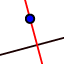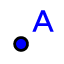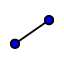# Copy of Angle Bisector with Incenter

Topic:
Angles

## Directions

1) Use the POLYGON toolto construct triangles A(2,3) B(6,13) and C(14,3) 2) Use the INTERSECTION toolto construct the Angle Bisector of each side 3) Use the POINT toolto construct the intersection of the 3 Angle Bisectors and record its coordinates on your worksheet. 4) Use the SEGMENT toolto construct the segment from the incenter to the sides and make sure the segments is perpendicular to the side.

## Questions

1)Is it ever possible for a triangle's INCENTER to lie OUTSIDE the triangle If so, under what condition(s) will this occur? 2) Is it ever possible for a triangle's INCENTER to lie ON the triangle itself? If so, under what condition(s) will this occur?